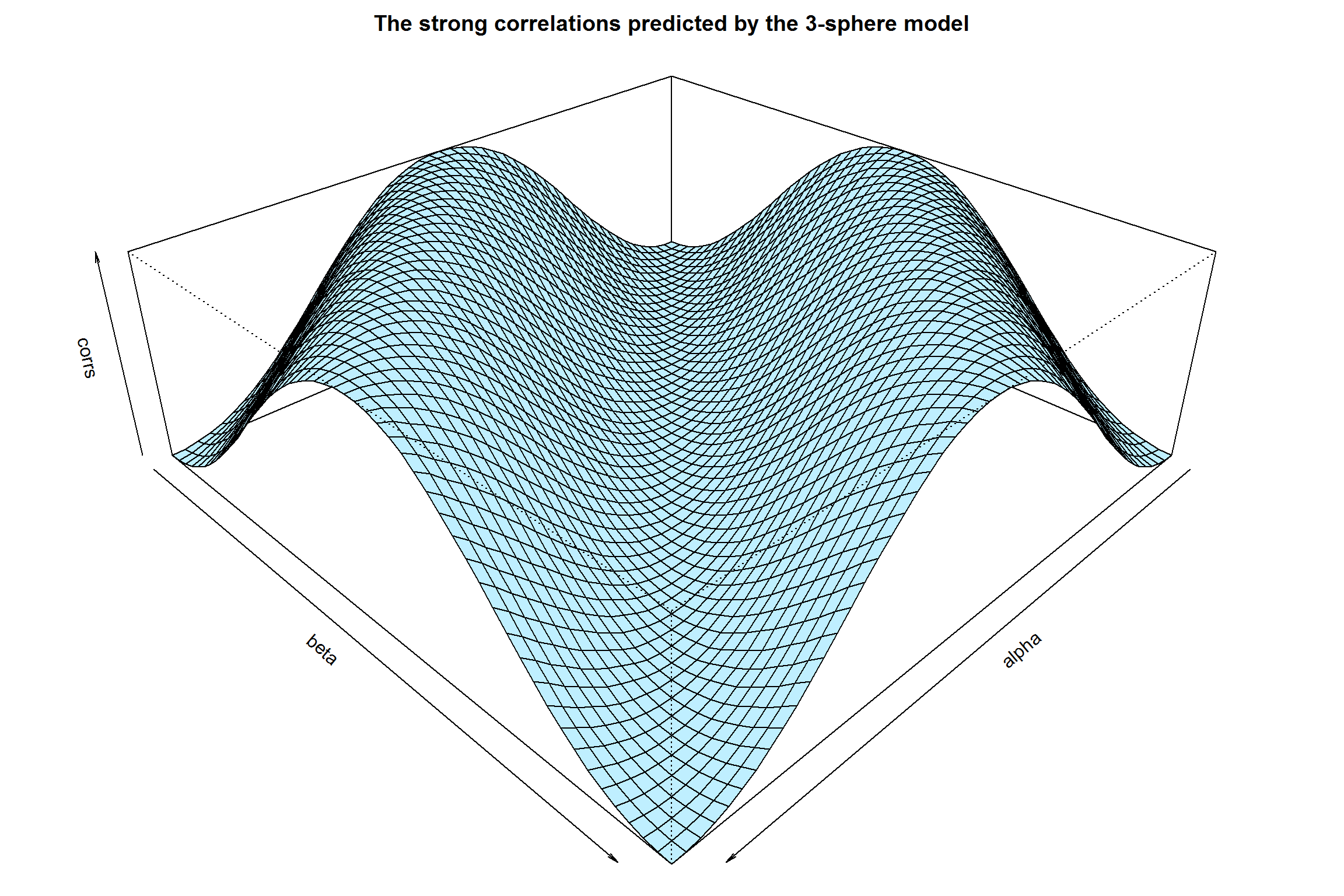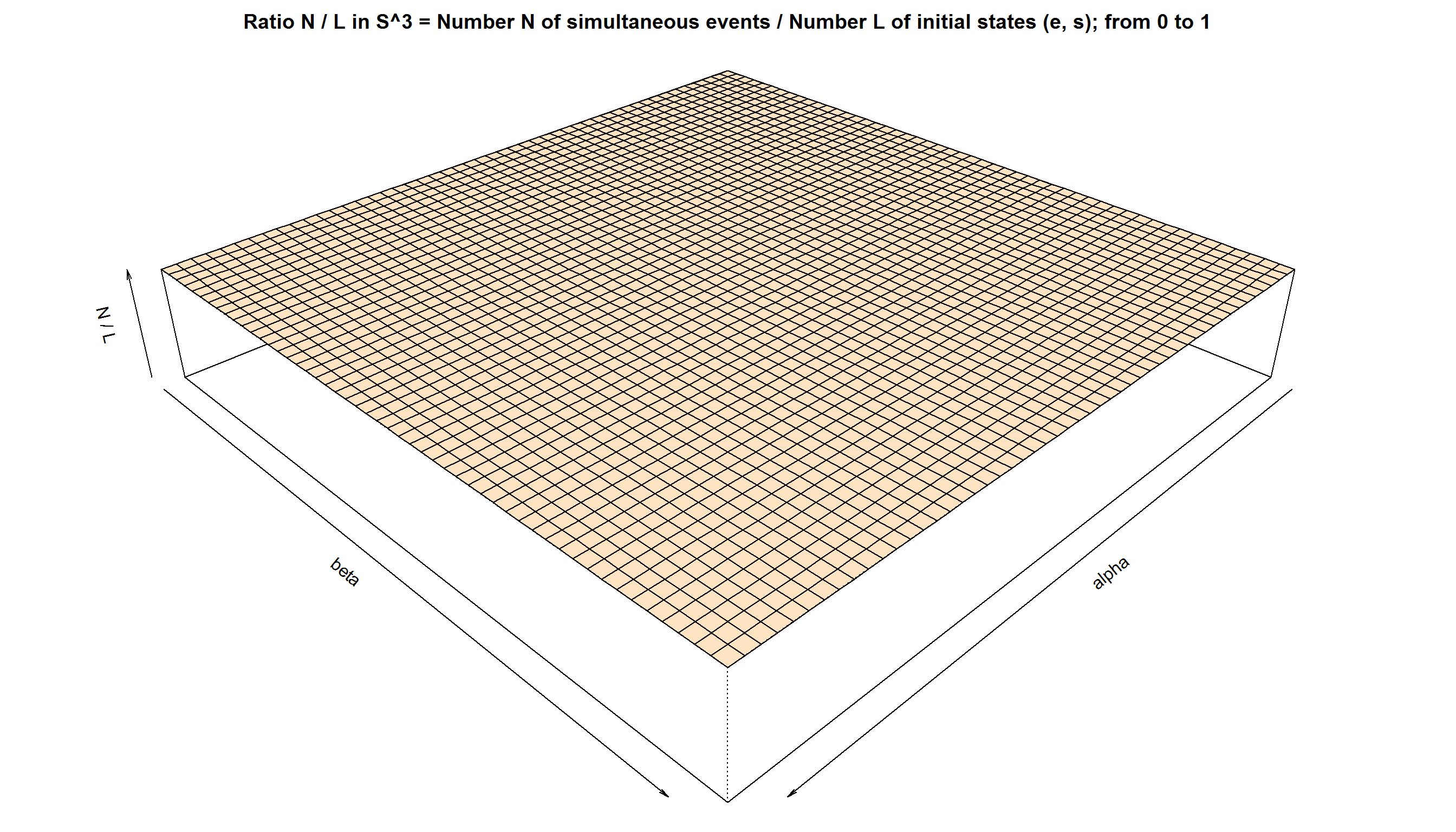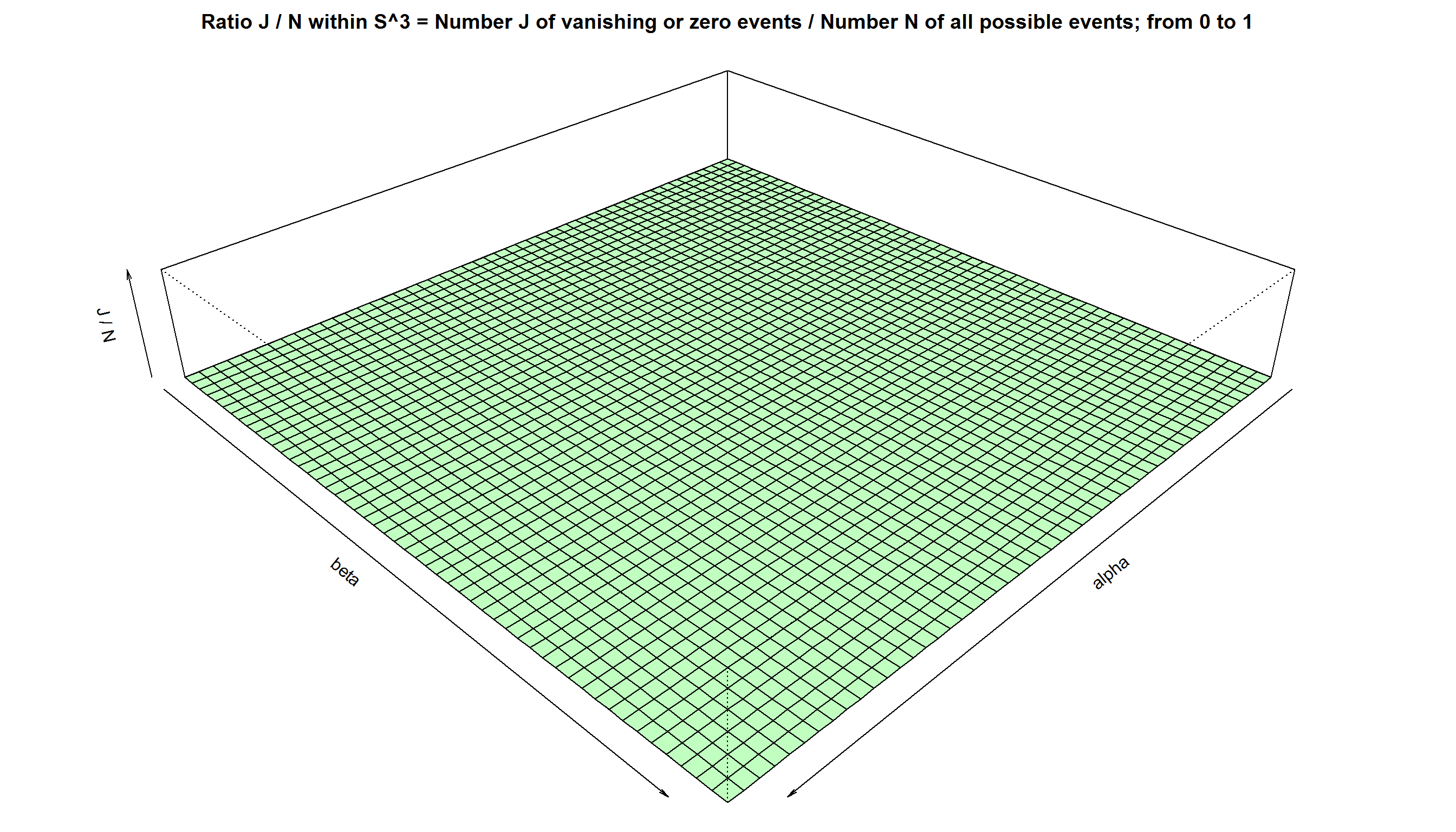# Metric Topology and the Origins of Quantum Correlation

by Joy Christian

Einstein Centre for Local-Realistic Physics, 15 Thackley End, Oxford OX2 6LB, United Kingdom

Publication date: 12 August 2015

# For a theoretical background, please see http://arxiv.org/abs/1405.2355

# and http://www.sciphysicsforums.com/spfbb1/viewtopic.php?f=6&t=188#p5129
Angles = seq(from = 0, to = 360, by = 7.2) * 2 * pi/360

K = length(Angles) # The total number of angles between 0 and 2pi

corrs = matrix(nrow = K, ncol = K, data = 0) # Container for correlations

Js  = matrix(nrow = K, ncol = K, data = 0) # Container for "zero" events

Ns  = matrix(nrow = K, ncol = K, data = 0) # Container for non-zero events

Ls  = matrix(nrow = K, ncol = K, data = 0) # Container for initial states
# A canvas of auxiliary pre-ensemble (analogous to pre-reduced phase space):

M = 10^4  # Size of the pre-ensemble. Next one can try 10^5, or even 10^6

r = runif(M, 0, 2*pi) # M uniformly distributed numbers between 0 and 2pi

z = runif(M, -1, +1) # M uniformly distributed numbers between -1 and +1

h = sqrt(1 - z^2)

x = h * cos(r)

y = h * sin(r)

e = rbind(x, y, z)  # A 3xM matrix, with M columns of e-vectors representing
# the x, y, z coordinates of uniformly distributed points on an S^2. In what
# follows these M number of pre-states will define an auxiliary pre-ensemble
# Defining the metrical and topological structures on S^3:

# So far we have set the variables in R^3. We now construct a metric on S^3

s = runif(M, 0, pi) # Initial states of the spins are the pairs (e, s) within S^3

f = -1 + (2/sqrt(1 + ((3 * s)/pi))) # For details see the paper arXiv:1405.2355

g = function(u,v,s){ifelse(abs(colSums(u*v)) > f, colSums(u*v), 0)}

# g(u,v,s) is non-vanishing only if |u.v| > f(s) ; g(u,v,s) --> "dot" as s --> pi

# Defines an inner product on S^3, thus changing the space from R^3 to S^3

# colSums(u * v) = u.v = the standard inner product between u and v in R^3

# u and v are orthogonal to each other in S^3 when abs(colSums(u * v)) < f

# The metric g(u,v,s) reduces to the standard Euclidean metric in R^3 for f --> 0
# Computing the "quantum" correlations:

for (i in 1:K) {
alpha = Angles[i]
a = c(cos(alpha), sin(alpha), 0)  # Measurement direction 'a'

for (j in 1:K) {
beta = Angles[j]
b = c(cos(beta), sin(beta), 0)  # Measurement direction 'b'

A = +sign(g(a,e,s))  # Alice's measurement results A(a, e, s) = +/-1

B = -sign(g(b,e,s))  # Bob's measurement results B(b, e, s) = -/+1

N = length((A*B)[A & B]) # Number of all possible events observed in S^3

corrs[i,j] = sum(A*B)/N  # Product moment correlation coefficient E(a, b)

# ----------------------------------------------------------------------

# Metric g(u,v,s) forbids the results (0,+), (0,-), (+,0), (-,0), & (0,0)

# Probabilities for (0,+), (0,-), (+,0), (-,0), and (0,0) vanish exactly

Cuu = length((A*B)[A > 0 & B > 0])   # Coincidence count of (+,+) events

Cdd = length((A*B)[A < 0 & B < 0])   # Coincidence count of (-,-) events

Cud = length((A*B)[A > 0 & B < 0])   # Coincidence count of (+,-) events

Cdu = length((A*B)[A < 0 & B > 0])   # Coincidence count of (-,+) events

# corrs[i,j] = (Cuu + Cdd - Cud - Cdu) / (Cuu + Cdd + Cud + Cdu) # = -a.b

# -----------------------------------------------------------------------

# There are no "0 outcomes" within S^3: Cou = Cod = Cuo = Cdo = Coo = 0

J = length((A*B)[g(a,e,s) & A == 0 & g(b,e,s) & B == 0]) # Number of null events

J = length((A*B)[g(a,e,s) & A == 0 | g(b,e,s) & B == 0]) # Number of null events

Js[i,j] = J # Total number of "vanishing" or "zero" events "observed" within S^3

Ns[i,j] = N # The total number of events simultaeously observed by Alice and Bob

# -------------------------------------------------------------------------------

# corrs[i] = sum(A)/length(A)  # Verifies < A > = 0 for all 'a' regardless of 'b'

# corrs[j] = sum(B)/length(B)  # Verifies < B > = 0 for all 'b' regardless of 'a'

# --------------------------------------------------------------------------

# Computing the same correlations with the standard Euclidean metric in R^3

C = sign(g(a,e,s))
D = sign(g(b,e,s))

o = x[C & D]
p = y[C & D]
q = z[C & D]

w = rbind(o,p,q) # N vectors in R^3 representing the initial states within S^3

a.w = colSums(a*w) # Standard inner product in R^3 between the vectors a and w
b.w = colSums(b*w) # Standard inner product in R^3 between the vectors b and w

# corrs[i,j] = sum(-sign(a.w)*sign(b.w))/N # Another way of calculating E(a, b)

L = length(w)/3  # The total number of initial states (e,s) emitted within S^3

Ls[i,j] = L  # Total number of initial particles (e,s) emitted from the source
}
}
par(mar = c(0, 0, 2, 0))
persp(x = Angles, y = Angles, main = "The strong correlations predicted by the 3-sphere model", z = corrs, zlim = c(-1, 1), col = "lightblue1", theta = 135, phi = 30, scale = FALSE, xlab = "alpha", ylab = "beta")par(mar = c(0, 0, 2, 0))
persp(x = Angles, y = Angles, main = "Ratio N / L in S^3 = Number N of simultaneous events / Number L of initial states (e, s); from 0 to 1", z = Ns/Ls, zlim = c(0, 1), col = "bisque", theta = 135, phi = 30, scale = FALSE, xlab = "alpha", ylab = "beta", zlab = "N / L")par(mar = c(0, 0, 2, 0))
persp(x = Angles, y = Angles, main = "Ratio J / N within S^3 = Number J of vanishing or zero events / Number N of all possible events; from 0 to 1", z = Js/Ns, zlim = c(0, 1), col = "darkseagreen1", theta = 135, phi = 30, scale = FALSE, xlab = "alpha", ylab = "beta", zlab = "J / N")The last plot can be used to verify that there are no zero outcomes regardless of the metric defining the physical space by the parameter “s”.

# Both CHSH and Clauser-Horne / Eberhard inequalities are violated in the 3-sphere model:
alpha <- 0 * pi/180
beta <- 45 * pi/180
a <- c(cos(alpha), sin(alpha), 0) # Measurement direction 'a'
b = c(cos(beta), sin(beta), 0)  # Measurement direction 'b'
A = +sign(g(a,e,s)) # Alice's measurement results A(a, e, s) = +/-1
B = -sign(g(b,e,s)) # Bob's measurement results B(b, e, s) = -/+1
C_0_45 = length((A*B)[A > 0 & B > 0]) # Coincidence count of (+,+) events
N_0_45 = length((A*B)[A & B]) # Coincidence count of all possible events
E_0_45 <- sum(A * B)/N_0_45

alpha <- 0 * pi/180
beta <- 135 * pi/180
a <- c(cos(alpha), sin(alpha), 0) # Measurement direction 'a'
b = c(cos(beta), sin(beta), 0)  # Measurement direction 'b'
A = +sign(g(a,e,s)) # Alice's measurement results A(a, e, s) = +/-1
B = -sign(g(b,e,s)) # Bob's measurement results B(b, e, s) = -/+1
C_0_135 = length((A*B)[A > 0 & B > 0]) # Coincidence count of (+,+) events
N_0_135 = length((A*B)[A & B]) # Coincidence count of all possible events
E_0_135 <- sum(A * B)/N_0_135

alpha <- 90 * pi/180
beta <- 45 * pi/180
a <- c(cos(alpha), sin(alpha), 0) # Measurement direction 'a'
b = c(cos(beta), sin(beta), 0)  # Measurement direction 'b'
A = +sign(g(a,e,s)) # Alice's measurement results A(a, e, s) = +/-1
B = -sign(g(b,e,s)) # Bob's measurement results B(b, e, s) = -/+1
C_90_45 = length((A*B)[A > 0 & B > 0]) # Coincidence count of (+,+) events
N_90_45 = length((A*B)[A & B]) # Coincidence count of all possible events
E_90_45 <- sum(A * B)/N_90_45

alpha <- 90 * pi/180
beta <- 135 * pi/180
a <- c(cos(alpha), sin(alpha), 0) # Measurement direction 'a'
b = c(cos(beta), sin(beta), 0)  # Measurement direction 'b'
A = +sign(g(a,e,s)) # Alice's measurement results A(a, e, s) = +/-1
B = -sign(g(b,e,s)) # Bob's measurement results B(b, e, s) = -/+1
C_90_135 = length((A*B)[A > 0 & B > 0]) # Coincidence count of (+,+) events
N_90_135 = length((A*B)[A & B]) # Coincidence count of all possible events
E_90_135 <- sum(A * B)/N_90_135
abs(E_0_45 - E_0_135 + E_90_45 + E_90_135)  # The absolute bound is supposed to be 2
##  2.851039
# Quantum mechanics and the 3-sphere model predict 2.8284 (up to statistical error)
R_0_45 = C_0_45 / N_0_45         # The rate of detecting (+,+) coincidence at (0, 45)

R_0_135 = C_0_135 / N_0_135      # The rate of detecting (+,+) coincidence at (0, 135)

R_90_45 = C_90_45 / N_90_45      # The rate of detecting (+,+) coincidence at (90, 45)

R_90_135 = C_90_135 / N_90_135   # The rate of detecting (+,+) coincidence at (90, 135)

R_a = 1/2 # Since < A > = 0 for all 'a' regardless of 'b', as verified above

R_b = 1/2 # Since < B > = 0 for all 'b' regardless of 'a', as verified above

(R_0_45 - R_0_135 + R_90_45 + R_90_135 - R_a - R_b) # The lower bound is supposed to be -1
##  -1.21093
# Quantum mechanics and the 3-sphere model predict -1.2071 (up to statistical error)